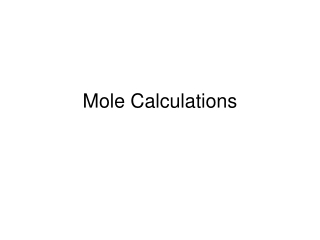DownloadDownload PresentationMole Calculations

# Mole Calculations

Download Presentation## Mole Calculations

- - - - - - - - - - - - - - - - - - - - - - - - - - - E N D - - - - - - - - - - - - - - - - - - - - - - - - - - -
##### Presentation Transcript

1. Mole Calculations

2. Mole Calculations

3. Mole Calculations MM = m/n n = m/M m = Mn m N = nNA n = N/NA n MM Factor Label! Let the units guide you

4. Determining Molar Mass Recall: Add the individual molar masses MM(NH4)3PO4 = 3(N + 4H) + P + 4O = 3N + 12H + P + 4O = 3(14.01) + 12(1.01) + 30.97 + 4(16.00) = 149.12 g/mol

5. MM = m/n n = m/M m = Mn Converting Mass to Moles Example: How many moles of calcium carbonate are there in 1.5 kg? n = m/MM = 1500 g  100.09 g/mol = 15 mol Given: m CaCO3 = 1500 g MM = 40.08 + 12.01 + (3 X 16.00) = 100.09 g/mol n = 1500 g X 1 mol 100.09 g = 15 mol

6. MM = m/n n = m/M m = Mn Converting Moles to Mass Example: What is the mass in grams of 0.346 mol of sodium sulfate? m = MMn = 142.04 g X 0.346 mol 1 mol = 49.1 g Given: n Na2SO4 = 0.346 mol MM = (2 X 22.99) + 32.06 + (4 X 16.00) = 142.04 g/mol

7. N = nNA Finding Number of Atoms or Molecules How many atoms of copper are there in 2.0 moles? N = nNA = 2.0 mol X 6.02 X 1023 atoms 1 mol = 1.2 X1024 atoms of copper Given: n Cu = 2.0 mol NA = 6.02 X 1023

8. Given: m = 1.00 X 103 g MM = (12 X 12.01) + (22 X 1.01) + (11 X 16.00) = 342.34 g/mol How many sugar (sucrose, C12H22O11) molecules are there in a 1.00 kg bag? n = 1.00 X 103 g X 1 mol 342.34 g = 2.921 mol N = nNA = 2.921 mol X 6.02 X 1023 molecules 1 mol = 1.76 X1024 molecules of sugar

9. How many carbon atoms are there in a 1.00 kg bag of sugar (sucrose, C12H22O11) ? N = 1.76 X 1024 molecules of sugar X 12 carbon atoms 1 molecule sugar = 2.11 X 1025carbon atoms

10. Mass (g) Concentration (mol/L) g/mol Vol   Moles (mol)  6.02 X 1023 22.4  # of molecules/atoms/ions Volume (L) at STP (0˚C, 101.3 kpa)

11. Homework • Finish Mole Worksheet • Change “volume” to “mass” in Q3 • P. 170 #7-13 • P. 171 #1-5 • P. 172-173#1-8